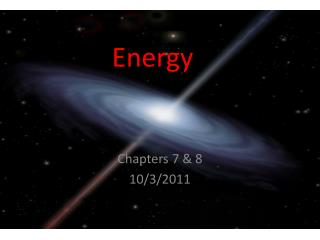DownloadDownload PresentationEnergy

# Energy

Télécharger la présentation## Energy

- - - - - - - - - - - - - - - - - - - - - - - - - - - E N D - - - - - - - - - - - - - - - - - - - - - - - - - - -
##### Presentation Transcript

1. Energy Chapters 7 & 8 10/3/2011

2. Potential + Kinetic = Total Energy WhereP.E = mghandK.E = ½ mV2and E = W = F xd Where Total Energy is conserved

3. Hooke’s Law • Mathematically: • Fspring = -kx • Where k = the spring constant in N/m • And x = (x – xequilibrium) in meters • Definition: • The force required to stretch/compress a spring is proportional to x (the amount of stretch/compression) • A “stiffer” spring will cause an increased spring constant, where a “looser” spring will decrease the spring constant

4. W = Force X distance for a constant force. For a changing force W = • W = Fdx = kxdx = kxdx • W = kx2/2 from xi to xf= potential energy • Since work = Force X distance, to hold a spring in place it requires no work, just force. Work is only done when the spring is moving!

5. Delta x oscillates in a sin wave as time goes on when a spring is released

6. For purely frictionless mechanical things that travel in straight lines PE & KE are constant so :delta PE (x) = - delta KE (x)

7. Conservative System • It doesn’t matter how (what path) you move in—as you move from higher potentials you gain kinetic energy and as you move from lower potentials you lose kinetic energy. • Friction makes a system not-conservative. You must factor in heat in this case • KE + PE + Heat = Total energy • (where KE & PE are mechanical energy)

8. Rate of Change of Energy • Delta Energy / delta time = power • OR Work / delta time (for strictly mechanical energy) = power • Power units is J/s = watt • Examples of power/energy relationships: • Heat takes a lot of energy, so it requires more watts (AC unit) whereas sound takes next to no energy and uses very little power (the stereo)

9. Examples • *The ball to the right is falling with an increasing speed. As its speed increases, its kinetic energy grows. Where is this energy coming from, since the laws of physics say that energy cannot be created or destroyed?

10. The increasing kinetic energy is coming from the initial gravitational potential energy and the potential energy as it falls toward the earth. As shown to the right, the ball begins with a potential energy of 600 J and 0 J of kinetic energy, but ends with 0 J of Potential energy and 600 J of Kinetic energy. No energy is created or destroyed

11. If a cannon sits at the top of a 50 m tall cliff and fires a cannonball into the air over the edge at an angle of 30 degrees and the initial velocity of the ball is 20m/s, what is the speed just before the cannon hits the ground? 20 m/s 30o ------------------- I 50m I I I I

12. Solving without Energy Vi=20m/s Yi=50m Yf=0m θ= 300 • Yf= yi + Vyit + ½ayt2 • 0 = 50m + 20sin30o + ½ (-9.8m/s2) t2 • 0 = 50m + 10m/s – 4.9m/s2 t2 • Quadratic Results: -2.3s & 4.4s • a t + Vxi = vyf • Vyf = (-9.8m/s2)(4.4s) + 20cos300 • Vyf = -32.8m/s • Vf = √(Vfx2 + Vfy2) • Vf= √(17.32 +32.92) • Vf = 37.1m/s Seems like a lot of math…

13. Solving Using Energy • ΣEi = ΣEf • GPEi + Kei = Kef • mgh + ½mvi2 = ½mvf2 • Vf = √(2gh + vi2) • Vf = √[(2)(9.8m/s2)(50m) + 20m/s] • Vf = 37.1m/s Easier right? Be careful, though this only works when the question does not ask for a direction

14. A 10 kg block is sliding down a ramp inclined at 200 from the ground. The block and ramp have a coefficient of kinetic friction of 0.2. The top of the ramp is 2 m above the ground. How much energy was lost to friction as the block slides down the ramp? What is the final speed of the block when it reaches the bottom?